# English Typing Lessons

# Typing Lesson Lesson Name Action
1 Mid Row Keys [A] [S] [D] [F] [J] [K] [L] [;]
2 Mid Row Keys [G] [H] [‘]
3 Top Row Keys [Q] [W] [U] [ I ]
4 Top Row Keys [E] [R] [O] [P] with suitable
5 Top Row Keys [T] [Y]
6 Bottom Row Keys [Z] [X] [M]
7 Bottom Row Keys [C] [V] [N]
8 Bottom Row Keys [B] {Tip Left Handed Person should use Right Hand Finger}
9 Bottom Row Keys Comma[,] and Dot[.]
10 Number Row keys    [/]
11 Number Row Keys       
12 Symbol Key 'Open and Close Bracket', 'Hyphen', 'Equal To'
13 Symbol Key Key Tilde and Backslash
14 Right Shift Shift Right Key with Q,W,E,R,T,A,S,D,F,G,Z,X,C,V,B to Capitalize
15 Left Shift Shift Left Key with Y,U,I,O,P,H,J,K,L,N,M to Capitalize
16 Special Symbols Symbol ~ ! @ # \$ %
17 Special Symbols Symbol ^ & * ( ) _ +
18 Special Symbols Symbol { } | : “ > < ?
19 All Key Combine Typing
Exercise Name {{this.exeDataAll.test_name}}
{{exeLetter}}Click to {{ this.status == 'paused' ? 'Resume' : 'Start'}}
{{ Math.round(this.f_speed)}}WPM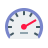Net Speed Gross Speed {{ Math.round(this.f_g_speed)}}WPM## Formula Used:Gross Speed = (Total Character Typed / 5) / Time Taken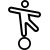Net Speed = Gross Speed - [(Wrong Character Typed / 5) / Time Taken]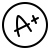Backspace, Space, Shift Keypress, Skipped Words are not counted for calculation.

# ANOP TYPING TUTOR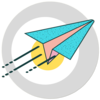{{ this.exerciseData.length }} Total Words
{{ this.c_Word + 1 }} Current Word
{{ this.f_g_speed>0 ? Math.round(this.f_g_speed) : '0' }} Gross Speed WPM
{{ Math.round(this.f_speed) }} Net Speed WPM
{{ Math.round(this.accuracy) }} Accuracy %
{{ this.time }}
{{ this.no_r_Word }} Words Right
{{ this.no_w_Word }} Words Wrong
{{ this.no_s_Word }} Words Skip
{{ this.no_r_Letter }} Letters Right
{{ this.no_w_Letter }} Letters Wrong
Tutorial Name {{this.dataProp.tutorial_name}}Click to {{ !this.running && this.status == 'running' ? 'Resume' : 'Start'}}
{{ Math.round(this.f_speed)}}WPMNet Speed Gross Speed {{ Math.round(this.f_g_speed)}}WPM## Formula Used:Gross Speed = (Total Character Typed / 5) / Time TakenNet Speed = Gross Speed - [(Wrong Character Typed / 5) / Time Taken]Backspace, Space, Shift Keypress, Skipped Words are not counted for calculation.
{{systemWord}} {{userWord}}
{{ this.var_lf_1 ? this.upCommingKey : "^" }} {{ this.var_lf_2 ? this.upCommingKey : "^" }} {{ this.var_lf_3 ? this.upCommingKey : "^" }} {{ this.var_lf_4 ? this.upCommingKey : "^" }} {{ this.var_rf_1 ? this.upCommingKey : "^" }} {{ this.var_rf_2 ? this.upCommingKey : "^" }} {{ this.var_rf_3 ? this.upCommingKey: "^" }} {{ this.var_rf_4 ? this.upCommingKey : "^" }}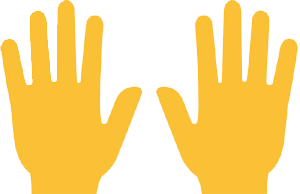~
`
! !
1
@ /
2
# रु
3
\$ +
4
% :
5
^
6
& -
7
*
8
( ;
9
) द्ध
0
_ ऋ़
. -
+
त्र =
Backspace
Tab
Q
q
W
w
E म्
e
R त्
r
T ज्
t
Y ल्
y
U न्
u
I प्
i
O व्
o
P च्
p
{ क्ष्
ख् [
} द्व
, ]
| द्य
? \
Caps
Lock
A
a
S
s
D क्
d
F थ्
f ि
G
g
H भ्
h
J श्र
j
K ज्ञ
k
L स्
l
: रू
;
" ष्
श् '
Enter
Shift
Z Z
z z
X ग्
x
C ब्
c
V
v
B
b
N
n
M
m
<
,
>
ण् .
? घ्
ध् /
Shift
Ctrl
Win
Alt
Space
Alt
Win
Ctrl

# ANOP TYPING TUTOR{{ this.tuteData.length }} Total Words
{{ this.tuteCursur + 1 }} Current Word
{{ this.f_g_speed>0 ? Math.round(this.f_g_speed) : '0' }} Gross Speed WPM
{{ Math.round(this.f_speed) }} Net Speed WPM
{{ Math.round(this.accuracy) }} Accuracy %
{{ this.time }}
{{ this.no_c_right }} Letters Right
{{ this.no_c_wrong }} Letters Wrong